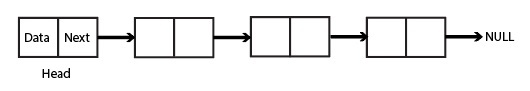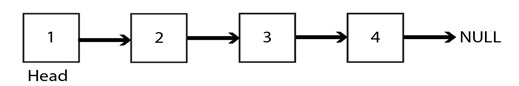# Delete a Linked List Using Recursion

A linked list is a linear data structure in which the elements are stored at non-contiguous memory locations. Each element consists of a node. A node is composed of a data field, which holds the value of the element, and an address field, which points to the location of the next node in the series.

The first node of the linked list is referred to as the ‘head’ of the list. The last element of the linked list can be defined as the element which points to NULL. A diagrammatic representation of a linked list is shown below.### Problem Statement

Given a linked list, the task is to delete all its elements using recursion, i.e. the head of the linked list should be NULL.

## Solution Approach

To delete all the elements of a linked list, we traverse through the linked list and delete all the nodes one by one. The linked list is considered empty when the head is equal to NULL.

### Alogorithm

The approach consists of the following steps −

• If the head equals NULL, then return

• Delete the node that the head node points to recursively.

### Pseudocode

Class node()

• Define public variable ‘data’

• Define public variable ‘next’

• Constructor Function node(val)

• Initialise data = val

• Initialise next = NULL

Function insert(key)

• Initialize new node ‘n’ with data value ‘key’.

• return

• While (temp->next != NULL)

• temp = temp->next

• Set temp->next = n

• return

Function deleteLL()

• return

Function printLL()

• while (temp != NULL)

• print temp->data

• temp = temp->next

• return

## Dry Run

Input: 1 -> 2 -> 3 -> 4
Output: [ ]


Explanation − After inserting all the nodes, one by one, in the linked list, the linked list can be represented asWhen the deleteLL() function is called with the head of this list (i.e., node 1), it first checks if the head is NULL. Since it's not, it calls itself with the next node in the list (i.e., node 2).

The second call to deleteLL() checks if the current head (i.e., node 2) is NULL. Since it's not, it calls itself with the next node in the list (i.e., node 3).

Similarly, it calls itself with the node 4. After this, in the next call, the head = NULL since we have reached the end of the linked list. So the function returns to the previous call in the linked list and frees up the current head node.

In simple words, the nodes are deleted in reverse order: 4 -> 3 -> 2 -> 1.

### Example: C++ Program

The following C++ program inserts a series of nodes, one by one, in the linked list using the insert() function. It then prints the original list using the function printLL(). After that it deletes all the nodes of the list by calling the function deleteLL() recursively. It deletes the nodes in reverse order. It then assigns head to NULL. Finally it prints the empty linked list using the function printLL().

The following C++ program recursively deletes the given linked list −

#include <bits/stdc++.h>
using namespace std;
// A linked list node class. Each node consists of a data field and an address field.
class node {
public:
int data;
node *next;

node(int val){
data = val;
next = NULL;
}
};

// This function inserts a node to form a linked list.
// We push a new node to the end of the list if the head of the linked list is given.
node *n = new node(key);
return;
}
while (temp->next != NULL){
temp = temp->next;
}
temp->next = n;
return;
}

// This function recursively deletes all the nodes of the linked list one by one.
return;
}
}

// This function prints all the nodes of the linked list.
while (temp != NULL){
cout << temp->data << " ";
temp = temp->next;
}
}

// Driver function
int main(){
cout << "Original Linked List: ";
printLL(head); // 1 2 30 4 5 60
cout << "Linked List after Deletion: ";

// after we free(head), it now points to an illegal location
// so we set head equal to NULL
return 0;
}


### Output

Original Linked List: 1 2 30 4 5 60 Linked List after Deletion:


## Time and Space Complexity Analysis

### Time Complexity: O(n)

• The insert() function has a time complexity of O(n) as it traverses the linked list to find the end.

• The printLL() function has a time complexity of O(n) as it also traverses the entire linked list.

• The deleteLL() function, which deletes the linked list recursively, has a time complexity of O(n) as it visits each node once.

### Space Complexity: O(n)

• The insert() function has a space complexity of O(1) because it does not require any additional space.

• Additionally, the printLL function also uses no extra space, giving it an O(1) space complexity.

• The deleteLL() function is a recursive function that uses the call stack to keep track of the nodes to be deleted. The maximum depth of the call stack would be equal to the number of nodes in the linked list. Therefore, the space complexity of the deleteLL function is O(n).

Therefore, the overall space complexity of the code is O(n).

## Conclusion

A linked list can be used to implement data structures like stacks, queues and hash tables. They are useful data structures and can be considered as dynamic arrays. This article discusses the recursive approach to delete a linked list. It explains the concept of a linked list, the approach to the recursive solution along with the C++ Program code, dry run and time and space complexity analysis in great detail.

Updated on: 19-Apr-2023

335 Views